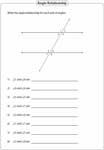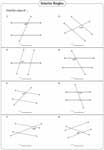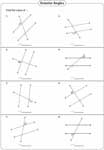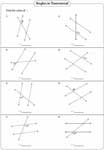# Angles Formed by a Transversal Worksheets

This page contains identifying the relationship between the angles formed by a transversal, finding the value of interior, exterior, alternate and same side angles (also called consecutive angles), solving unknown variable using the given information and more. Easy level requires the direct answer. Moderate and Difficult levels require equation solving skill to solve the problems.

Angle Relationship: Interior and Exterior

Find the angle relationship between each pair of angles. It contains alternate interior, alternate exterior, same side interior and same side exterior.Angle Relationship in a Transversal

Write the angle relationship for each pair of angles formed by a transversal line. It contains interior angles, exterior angles, vertical angles, corresponding angles and linear pair.Finding Interior Angles

Each question contains parallel lines and a transversal. Using the interior angle theorem, find the value of x.Easy:

Moderate:

Difficult:

Finding Exterior Angles

In exterior angle, there are two types, alternate exterior and same side exterior. Apply exterior angle theorem and solve for x.Easy:

Moderate:

Difficult:

Finding Alternate and Same Side Angles

Using the properties of alternate and same side angles (consecutive angles), solve for the unknown variables.Easy:

Moderate:

Difficult:

Supplementary and Congruent Angles

Linear pair and same side angles are supplementary. Alternate angles, corresponding angles and vertical angles are congruent angles. These worksheets help review this concept.Angles in Transversal: Mixed Review

These worksheets give the complete review in practicing angles formed by a transversal.Easy:

Moderate:

Difficult:

The whole batch of angles formed by a transversal worksheets can be downloaded instantly.

Related Worksheets